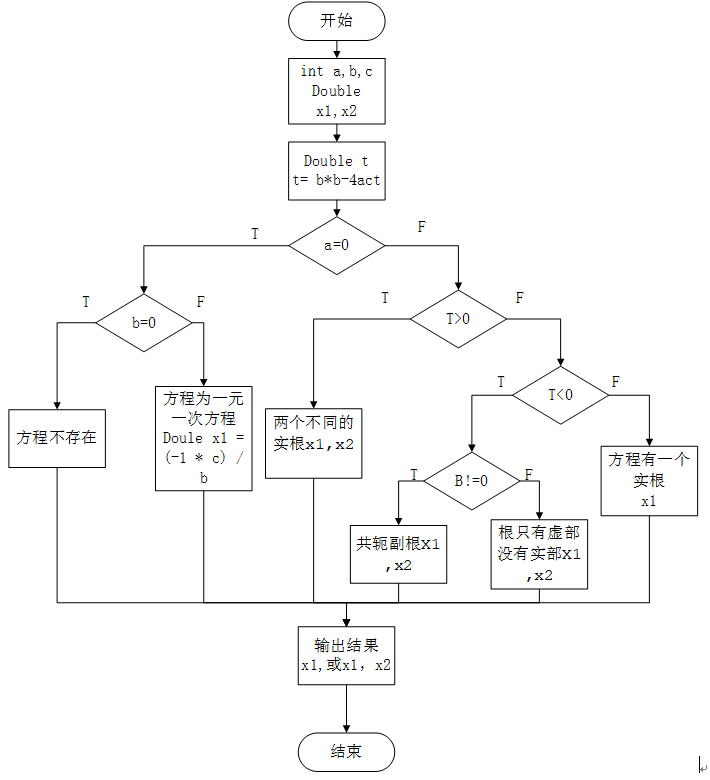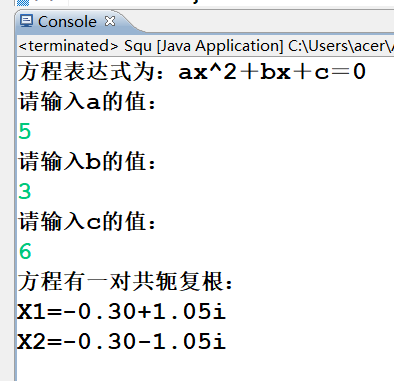# 求一元二次方程:ax2+bx+c=0的根，a、b、c由键盘输入。

## 流程图：## 程序代码：

package com.Squ.www; //创建一个Squ包

import java.util.Scanner;//导入java.util包中的Scanner类(使用此类可以方便的完成输入流的输入操作)
import java.math.BigDecimal;//导入java.math包中的BigDecimal(用来对超过	16位有效位的数进行精确的运算)

//对一元二次方程中各系数的不同情况作讨论，列出不同情况下根的分布，最终求出存在的根
public class Squ { //定义一个公共类---Squ
public static void main(String[]args)
{
double X1,X2;//定义两个解
System.out.println("方程表达式为：ax^2＋bx＋c＝0");//显示台输出---方程表达式为：ax2＋bx＋c＝0
Scanner sc=new Scanner(System.in);//用Scanner类获取控制台的输入(即获得从键盘输入的数)
System.out.println("请输入a的值：");//显示台输出提示---输入a的值
//每一次从键盘输入值后回车执行后面的内容
double a=sc.nextDouble();//接受控制台输入的数字给形参a赋值
System.out.println("请输入b的值：");//显示台输出提示---输入b的值
double b=sc.nextDouble();//接受控制台输入的数字给形参b赋值
System.out.println("请输入c的值：");//显示台输出提示---输入c的值
double c=sc.nextDouble();//接受控制台输入的数字给形参c赋值
double t=b*b-4*a*c;//定义一个变量t,且t=b*b-4*a*c,此时t为方程根的判别式

//用判断语句对方程的系数的不同情况作讨论，并得出根的分布情况
//① 判断当a、b、c都为0时，方程变为等式0=0;方程不存在！
if(a==0 && b==0 && c==0)
{
System.out.println("方程不存在！");//显示台输出
}
//② 当a、b都为0，而c不为0时，方程变为等式c=0,此中不含未知数，方程不存在！
else if(a==0 && b==0 && c!=0)
{
System.out.println("方程不存在！");//显示台输出
}
//③ 当a为0，且b、c不为0时,方程变为一元一次方程bx+c=0,此时方程只有一个解
else if(a==0 && b!=0 && c!=0)
{
System.out.println("此方程为一元一次方程");//显示台输出
double x1 = (-1 * c) / b;//定义一个变量result存放方程的解;此时解为：-c/b
System.out.println("方程的解为：" + resetValue(x1));
}
//④ 以上定义了t=b*b-4*a*c，若t>0,则t的开方为实数，此时方程有两个不同的实根，且两实根为相反数
else if(t>0)
{
System.out.println("方程有两个实根");//显示台输出
//第一根的算法：((-b) + Math.sqrt(t)) / 2 * a( Math.sqrt是指math方法中的sqrt类，即开方)
X1= ((-b) + Math.sqrt(t)) / 2 * a;
//第一根的算法：((-b) - Math.sqrt(t)) / 2 * a
X2= ((-b) - Math.sqrt(t)) / 2 * a;
//显示台输出两根的值
System.out.println("X1="+resetValue(X1));
System.out.println("X2="+resetValue(X2));
}
//⑤ 如果t<0,则t开方后产生虚数，此时方程有一对共轭副根
else if(t<0)
{
//⑥ 如果b不等于0，则其根为共轭副根，根由虚部和实部组成
if(b!=0)
{
X1=(-1*b)/(2*a);//根的实部
X2=Math.sqrt(-1*t)/(2*a);//根的虚部
System.out.println("方程有一对共轭复根：");//显示台输出
//输出时toString()表示用科学计数法输出根的值;加i指虚部的表示法;其中的"+、-"连接根的实部和虚部，形成共轭
System.out.println("X1="+resetValue(X1).toString()+"+"
+resetValue(X2).toString()+"i");//输出第一个根的值
System.out.println("X2="+resetValue(X1).toString() + "-"
+ resetValue(X2).toString() + "i");//输出第二个根的值
}
//⑦ 若b=0,则此时根只有虚部没有实部，是一对共轭复根，实部为0
else
{
X1=Math.sqrt(-1*t)/(2*a);//根中只有虚部
System.out.println("方程有一对相反虚根：");//显示台输出
//输出时toString()表示用科学计数法输出根的值;加i指虚部的表示法;其中的"+、-"连接根的实部和虚部，形成共轭，此时实部为0
System.out.println("X1=0-"+resetValue(X1).toString()+"i");
System.out.println("X2=0+"+resetValue(X1).toString()+"i");

}
}
//⑧ 若t=0,则方程有一个实根
else if(t==0)
{
X1=(-1*b)/2*a;//根的计算
System.out.println("方程有一个实根");//显示台输出
System.out.println("方程的解为："+resetValue(X1));//显示台输出方程的解
}

}

//使用静态方法将定义的值由double类型转换为BigDecimal类型(声明)
private static BigDecimal resetValue(double n) {
// TODO 自动生成的方法存根
BigDecimal bd=new BigDecimal(n);//将n的值赋给形参bd
//返回的值保留两位小数，默认用四舍五入方式 （ROUND_HALF_UP表示遇5进1；setScale规定要保留的小数位数）
return bd.setScale(2,BigDecimal.ROUND_HALF_UP);
}

}


## 程序运行结果图：02-171028
01-24457
04-042万+
03-311万+
09-074695
02-02327
04-20168
10-295175
10-064968
08-288698
01-161086
01-24718
09-161591
04-082万+
03-161万+
12-119706